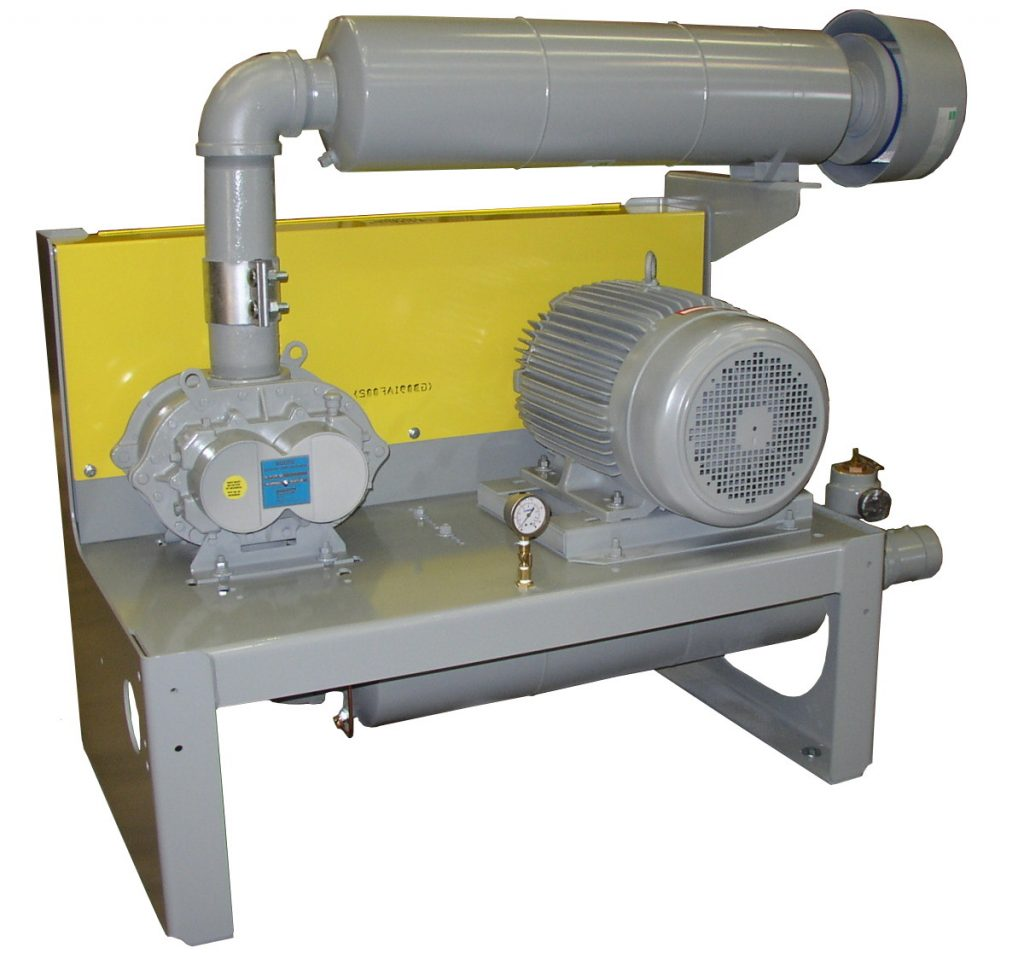# Blower and Vacuum Pump Heat Rejection Calculation

When a positivie displacement blower or vacuum pump package are installed inside a building, the following factors must be considered for the design of the room ventilation system. Inadequate or lack of room ventilation will create excessive temperatures around the blower or vacuum pump, motor and accessories leading to equipment failure.Heat rejection from the package:
The package will reject about 15 to 20% of the motor BHP into the building. This consists of motor heat loss, V-belt loss, blower radiation loss, silencer radiation loss and heat radiated from accessories.

PKHL=Package Heat Loss in Btu/Min = 0.2*Motor HP*42.4 =8.48*Motor HP

Piping Heat Loss:
The discharge piping inside the building will radiate heat inside the room.

PHL= Piping heat loss in Btu/Min= A*Y*( T24– T14)
A= Piping surface area in Sq.Ft.
Y= Radiation Constant = 2.855×10-11 Btu/Sqft/Min/R4
T2 = Piping surface temperature in Rankine
T1 = Room temperature in Rankine

Total Heat Load= PKHL+PHL = W*Cp* Δt

W= Ventilation air flow in Lb/Min
Cp= Specific heat for air = .24 Btu/Lb .°R
Δt= Room temperature rise above outside temperature in °F
V= Ventilation air flow in CFM = W/ ρ , where ρ is air density in Lb/Cubic Ft

Room ventilation system should be sized to keep the room temperature no more than 5ºF above maximum outside temperature of 100ºF. If outside temperature exceeds 100ºF, special motor insulation will be required.

Piping heat loss could be reduced by lagging the piping with insulation.

On vacuum service, do not discharge blower exhaust inside the building, pipe the exhaust to the outside.

It is also recommended that the exposed discharge piping be lagged with insulation to reduce noise inside the building. Package noise guarantee does not include noise from the external inlet/discharge piping supplied by others.

Example- Ventilation Air Flow Determination
Motor BHP = 100
Outside Temperature = 95°F = 460+95= 555ºR
Room Temperature = 100°F= 460+100= 560°R
Blower Discharge Temperature = 280°F= 460+280= 740°R
Discharge Pipe= 6″ diameter, 30 Feet long.
Surface area A= 3.14*(6/12)*30 = 47.1 Sq.Ft
Package Heat Loss = 8.48*100= 848 Btu/Min
Piping Heat Loss= 47.1*2.855×10-11*(7404-5604) = 293 Btu/Min
Total Heat Load = 848+293= 1141 Btu/Min
W = 1141/.24/5 = 950.8 Lb/Min
At sea level and at 95° F, Density of air ρ = 14.7*144/53.3/555= .071 Lb/Cubic ft

Ventilation Air Flow = 950.8/.071 = 13,391 CFM

For multiple packages installed in a room, add up the heat load from each package and piping losses to calculate required ventilation flow.